## 题目描述（中等难度）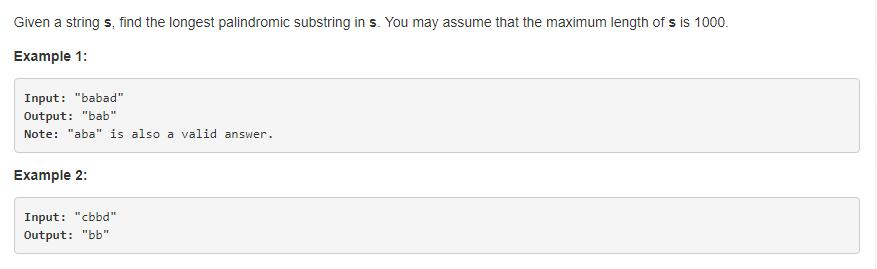## 解法二 最长公共子串

https://blog.csdn.net/u010397369/article/details/38979077

https://www.kancloud.cn/digest/pieces-algorithm/163624

arr [ i ][ j ] = arr [ i - 1 ][ j - 1] + 1 。

arr [ i ][ j ] 保存的就是公共子串的长度。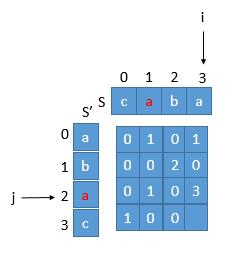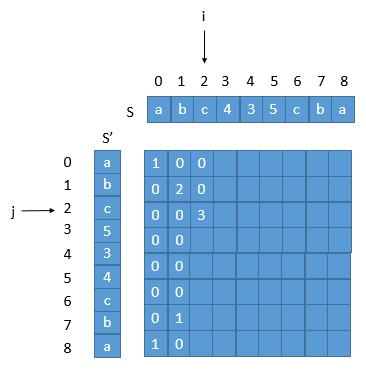## 解法三 暴力破解优化

$$P(i,j)=\begin{cases}true& \text{s[i,j]是回文串} \\false& \text{s[i,j]不是回文串}\end{cases}$$

$$P(i,j)=(P(i+1,j-1)\&\&S[i]==S[j])$$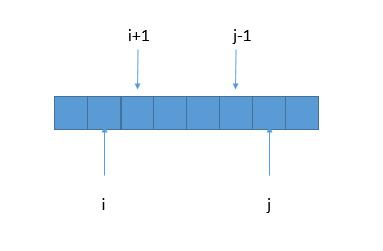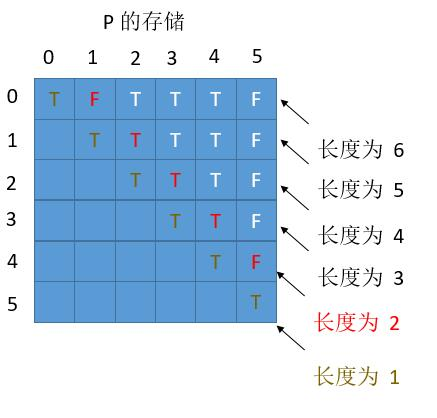$$P(i,j)=\begin{cases}true& \text{s[i,j]是回文串}\\false& \text{s[i,j]不是回文串}\end{cases}$$

$$P(i,j)=(P(i+1,j-1)\&\&S[i]==S[j])$$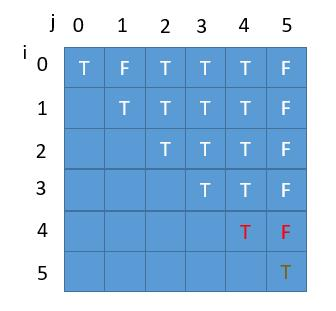## 解法四 扩展中心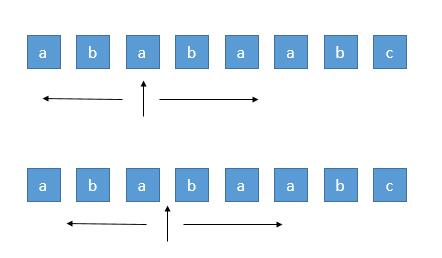## 解法五 Manacher’s Algorithm 马拉车算法。

https://segmentfault.com/a/1190000008484167

https://blog.crimx.com/2017/07/06/manachers-algorithm/

http://ju.outofmemory.cn/entry/130005

https://articles.leetcode.com/longest-palindromic-substring-part-ii/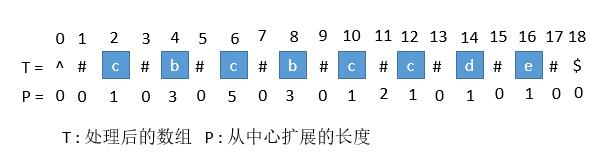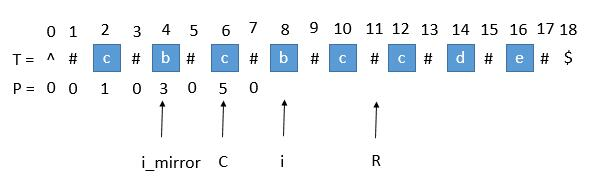### 超出了 R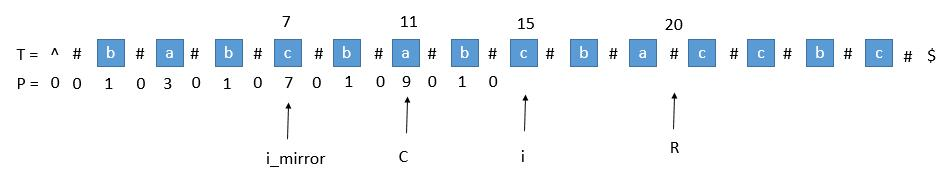### P [ mirror ] 遇到了左边界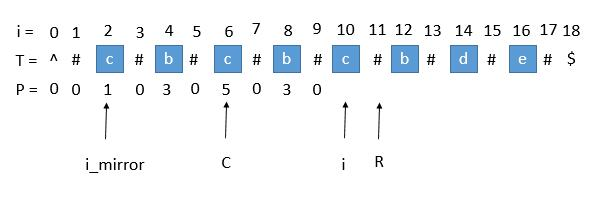### i 等于了 R## 总结